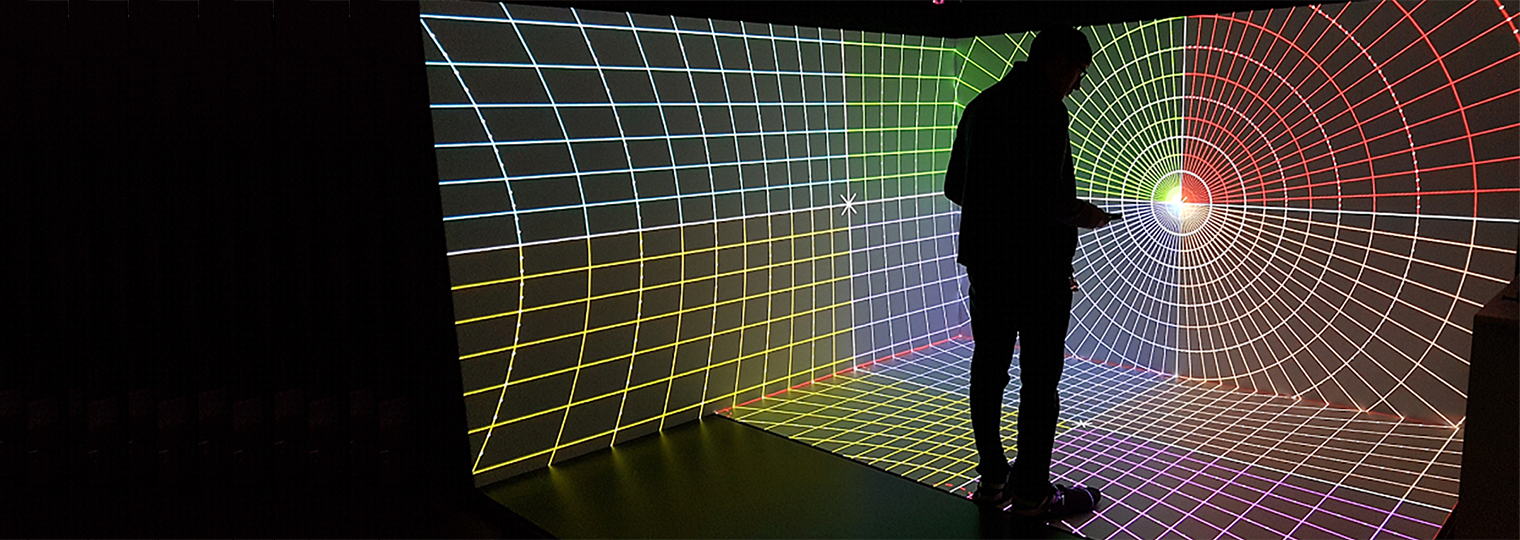# nDisplay的投影策略

## nDisplay中的投影策略

` "screen"`

`"camera" `

`"manual" `

`"mesh" `

` "picp_mesh"`

Picp_mesh

`"mpcdi"`

MPCDI

` "picp_mpcdi"`

PICP_MPCDI

` "easyblend"`

Easyblend

## 简单策略（将改名为"屏幕"策略）

``````[screen] id="scr_demo" loc="X=2,Y=0,Z=0" rot="P=0,Y=0,R=0" size="X=1,Y=1"
[viewport] id="vp_demo" x="0"  y="0"  width="800" height="800" projection="proj_simple_demo"
[projection] id="proj_simple_demo" type="simple" screen="scr_demo"``````

## 摄像机策略

``````[viewport] id="vp_demo" x="0"  y="0"  width="800" height="800" projection="proj_camera_demo"
[projection] id="proj_camera_demo" type="camera"``````

## 手动策略

### 案例1：自定义矩阵中的视锥

``````[viewport] id=vp_1 x=0   y=0   width=1000  height=600 projection=proj_manual_1
[viewport] id=vp_2 x=0   y=0   width=1000  height=600 projection=proj_manual_2
[projection] id=proj_manual_1 type="manual" rot="P=0,Y=0,R=0" matrix_left="[0.5 0 0 0] [0 0.999999 0 0] [1 0 0 1] [0 0 1 0]" matrix_right="[0.500001 0 0 0] [0 1 0 0] [-1 0 0 1] [0 0 1 0]"
[projection] id=proj_manual_2 type="manual" rot="P=0,Y=0,R=0" matrix="[0.500001 0 0 0] [0 1 0 0] [-1 0 0 1] [0 0 1 0]"``````

### 案例2：自定义角的视锥

``````[viewport] id=vp_1 x=0   y=0   width=1000  height=600 projection=proj_manual_1
[viewport] id=vp_2 x=0   y=0   width=1000  height=600 projection=proj_manual_2
[projection] id=proj_manual_1 type="manual" rot="P=0,Y=0,R=0" frustum_left="l=-15 r=0 t=10 b=-10" frustum_right="left=0 right=15 top=10 bottom=-10"
[projection] id=proj_manual_2 type="manual" rot="P=0,Y=0,R=0" frustum="l=0 r=15 t=10 b=-10"``````

## 基于网格体和PICP_Mesh

• 一种是通用型策略，称为 `"网格体（mesh——"`

• 一种用于摄象机内特效（In Camera VFX），称为 `"picp_mesh"`Picture in Camera Perspectivepicp））

• 相比 PFM（便携式浮动贴图）工作流，现在可以直接指定网格体，以便有效地扭曲渲染输出。

### 一般用途

*` [projection] id="proj_picpmesh_right" type="mesh" `

• 扭曲网格体的源由指定给具有"网格体"投影集视口的SceneMeshComponent引用表示：

• `[viewport] id="warped_viewport" x="0" y="0" width="1152" height="960" projection="proj_picpmesh_right"`

• 用于扭曲映射的UV信道0。

## 摄象机内特效

• 基于网格体的"picp_mesh"扭曲策略专为摄像机内特效（IncameraVFX）的视锥渲染而创建。

• `[projection] id="proj_picpmesh_up" type="picp_mesh" `

• 对于网格体分配，应使用PICP Module API，而非DisplayClusterProjectionAPI。

## MPCDI 和 PICP_MPCDI

MPCDI（多投影通用数据交换） 标准由"VESA多投影仪自动校准"（MPAC）任务小组开发。这是投影校准系统与多显示器配置中的设备进行通信的标准数据格式。

MPCDI被行业中的内容生产商和供应商广泛使用，例如：

• Scalable Display Technologies

• VIOSO

• Dataton Watchout

• 7thSense Design

### 使用.mpcdi文件

[projection] id=proj_mpcdi_demo type="mpcdi" file="D:\config.mpcdi" buffer="Mosaic" region="displayOrigin" origin=mpcdi_origin

### 明确规范

[projection] id="proj_mpcdi_demo" type="mpcdi" pfm="geom_left.pfm" alpha="geom_left_a.png" beta="geom_left_b.png" scale=1 origin=stage_origin

## EasyBlend（可扩展显示）

EasyBlend 校准数据的集成工作通过 Scalable SDK 完成，该SDK启用了扭曲/混合/梯形失真功能。这满足了使用多投影仪在非平面和复杂显示表面（例如曲面或圆顶形表面）上显示的要求。

Scalable Display Technologies 是一家专注于为复杂投影系统开发软件和SDK的公司。他们的SDK旨在通过扭曲和混合技术，为单幅图像的放大显示提供解决方案。考虑到Scalable Display Technologies已经有了现成的EasyBlend解决方案，并且可以处理大幅图像的扭曲和混合效果，因此我们选择将其集成到虚幻引擎中。

``````[projection] id=proj_easyblend_1 type="easyblend" file="E:\LocalCalibrationFlat\ScalableData.pol" origin=easyblend_origin_1 scale=0.1
[projection] id=proj_easyblend_2 type="easyblend" file="E:\LocalCalibrationFlat\ScalableData.pol_1" origin=easyblend_origin_1 scale=0.1
[projection] id=proj_easyblend_3 type="easyblend" file="E:\LocalCalibrationFlat\ScalableData.pol_2" origin=easyblend_origin_1 scale=0.1``````